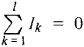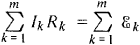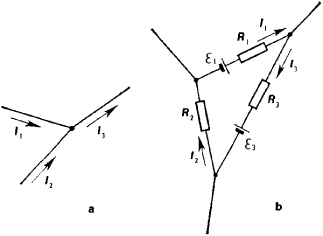# Kirchhoff's laws

Also found in: Dictionary, Thesaurus, Wikipedia.
Related to Kirchhoff's laws: Kirchhoff's circuit laws

## Kirchhoff's laws

[for Gustav R. KirchhoffKirchhoff, Gustav Robert
, 1824–87, German physicist. He served as professor of physics at the universities of Breslau (1850–54), Heidelberg (1854–74), and Berlin (from 1875). He is known especially for his work with the spectroscope in association with R. W.
], pair of laws stating general restrictions on the current and voltage in an electric circuitelectric circuit,
unbroken path along which an electric current exists or is intended or able to flow. A simple circuit might consist of an electric cell (the power source), two conducting wires (one end of each being attached to each terminal of the cell), and a small lamp (the
. The first of these states that at any given instant the sum of the voltages around any closed path, or loop, in the network is zero. The second states that at any junction of paths, or node, in a network the sum of the currents arriving at any instant is equal to the sum of the currents flowing away.
The following article is from The Great Soviet Encyclopedia (1979). It might be outdated or ideologically biased.

## Kirchhoff’s Laws

(Kirchhoff’s circuit rules), laws establishing the relationships of currents and voltages in branching electric networks carrying direct or quasi-stationary currents. The laws were formulated by G. R. Kirchhoff in 1847.

Kirchhoff’s first law follows from the law of conservation of electric charge. It states that the algebraic sum of currents Ik converging at any junction point (node) is zero; that is,where l is the number of currents converging at a given node. In this case, currents flowing toward the node are assumed to be positive, and the currents away from the node negative (Figure l,a). Kirchhoff’s second law states that in any closed path arbitrarily isolated from a complex network of conductors (Figure l,b), the algebraic sum of all voltage drops IkRk in the individual sections of the path (branch) is equal to the sum of electromotive forces (emf’s) Ƹk in this path; that is,where m is the number of branches in the closed path (in Figure l, m = 3) and Ik and Rk are the current and resistance of the branch numbered k. Here, a positive direction of currents and emf’s should be selected; for example, consider them to be positive if the direction of current coincides with tracing of the path in a clockwise direction and the emf increases the potential in the same direction of tracing and consider them negative in the opposite direction. Kirchhoff’s second law is obtained by applying Ohm’s law to the individual branches of a closed path.Figure 1

Kirchhoff’s laws make it possible to quantitatively analyze complex electric networks. For instance, strength and direction of current can be determined for any part of a branched system of conductors if the resistances and the emf’s are known for all of its branches. For a system of n conductors forming r nodes, n equations are set up. The r – 1 equation for nodes is based on Kirchhoff’s first law (the equation for the last node is not independent but is determined by the foregoing equations), and the n – (r – 1) equations for independent closed circuits are based on Kirchhoff’s second law. Each of the n conductors has to be entered in these latter equations at least once. Since in setting up equations it is necessary to take into account the directions of currents in conductors, which are not known in advance (and must be found by solving the system of equations), these directions are at first given arbitrarily. If during the solution for any current a negative sign is obtained, then this indicates that the direction of the current is opposite to the direction first assumed.

### REFERENCES

Frish, S. E., and Timoreva, A. V. Kurs obshchei fiziki, 7th ed., vol. 2. Moscow, 1958. Subsection 169.
Kalashnikov, S. G. Elektrichestvo. Moscow, 1956. (Obshchii kurs fiziki, vol. 2) Subsection 79.
References in periodicals archive ?
Physics is in a diffcult position relative to Kirchhoff's law, since the modern relationship between radiation and absorption, under equilibrium conditions, is based upon this work.
Planck's derivation of Kirchhoff's law, as presented in The Theory of Heat Radiation [9; pp.
Unfortunately, there is a difficulty at the very beginning of the Planck's elucidation of Kirchhoff's law.
This will lead directly to Kirchhoff's law. If Planck had properly weighed that the total radiation coming from the element d[tau] was equal to the sum of its emission and reflection, he would have started with dt d[tau] d[OMEGA] dv 2([[epsilon].sub.v] + [[rho].sub.v]), which would not lead to universality.
Through the exposition of Kirchhoff's law, we have been able to highlight that universality does not hold in cavity radiation.
The physics community has persisted in upholding Kirchhoff's law of thermal emission even though it has been refuted both recently [23-25] and in the past (see  for a discussion of the controversy surrounding Kirchhoff's law).
On the validity of Kirchhoff's law of thermal emission.
A critical analysis of universality and Kirchhoff's Law: a return to Stewart's law of thermal emission.
On the validity of Kirchhoff's law for a freely radiating body.
On the validity of Kirchhoff's law of heat radiation for a body in nonequilibrium environments.

Site: Follow: Share:
Open / Close